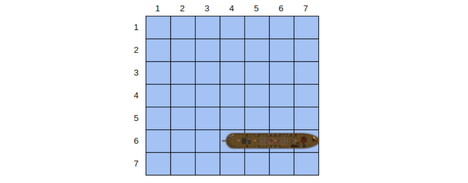# Practice on Toph

Participate in exhilarating programming contests, solve unique algorithm and data structure challenges and be a part of an awesome community.

# Find the Ship

You will be playing an interactive game in this problem.

The game is played on a $7\times 7$ square grid. Each cell of the grid is numbered with a pair $(r_i,c_i)$, where $r_i$ and $c_i$ are the row and column number $(1\leq r_i, c_i \leq 7)$.

The grid is covered with water and there is a ship inside it. The ship covers exactly 4 consecutive cells of the grid (either vertically or horizontally).
Check the picture below to get a clear idea:In this figure, the ship is located on cell $(6,4)$ to cell $(6,7)$.

Initially, you don't have any information about the grid. You have to find the location of the ship by performing the following operations:

• You can ask whether a cell $(r, c)$ contains some part of the ship. To do it, print a line in the following format:
? r c


In response, you will read a single character S or W, where S means the cell $(r, c)$ contains some part of the ship and W means it contains water.
There will be a limitation on the number of queries you can perform, see the subtasks portion for more details.

• You have to end the interaction by printing the ship location. To do it, print a line in the following format:
! r1 c1 r2 c2


Here, $(r1, c1)$ and $(r2, c2)$ are the cells that contain the two endpoints of the ship. You can print them in any order.

## Input

In the beginning, you will read an integer $T (1\leq T\leq 10000)$, the number of test cases. Interaction for the first test case will start right after that.

You will read one of the following as a response of query operation (details are already mentioned above):

• S
• W

• Subtask 1 (35 points): Perform no more than 13 queries for each case.
• Subtask 2 (65 points): Perform no more than 12 queries for each case.

In each subtask, You will get a "Wrong Answer" verdict if you use more queries than mentioned.

## Output

There are two types of output (details are already mentioned above):

1. ? r c
2. ! r1 c1 r2 c2

After printing the ship location, interaction for the next test case will start (if there is any).

Here is what a possible interaction would look like:

> 2
< ? 1 1
> S
< ? 1 2
> S
< ! 1 1 1 4
< ? 1 1
> W
< ? 1 2
> S
< ? 1 3
> W
< ! 1 2 4 2


>indicates what your program reads and < indicates what your program writes. These symbols are here to make it easy to understand. You do not have to print such symbols from your program.

•Interactive

### Statistics

43% Solution Ratio

lelbabaEarliest, 1M ago

lelbabaFastest, 0.2s

shahed95Lightest, 164 kB

shahed95Shortest, 1698B

### Submit